# E-PolyLearning

 1. Select the figure which satisfies the same conditions of placement of the dots as in Figure-X.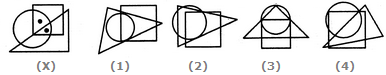a. 1 b. 2 c. 3 d. 4

 2. Select the figure which satisfies the same conditions of placement of the dots as in Figure-X.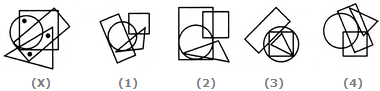a. 1 b. 2 c. 3 d. 4
 3. Select the figure which satisfies the same conditions of placement of the dots as in Figure-X.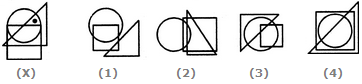a. 1 b. 2 c. 3 d. 4
 4. Select the figure which satisfies the same conditions of placement of the dots as in Figure-X.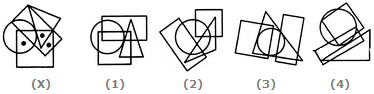a. 1 b. 2 c. 3 d. 4
 5. Select the figure which satisfies the same conditions of placement of the dots as in Figure-X.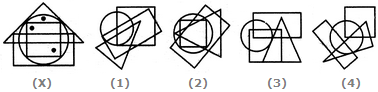a. 1 b. 2 c. 3 d. 4